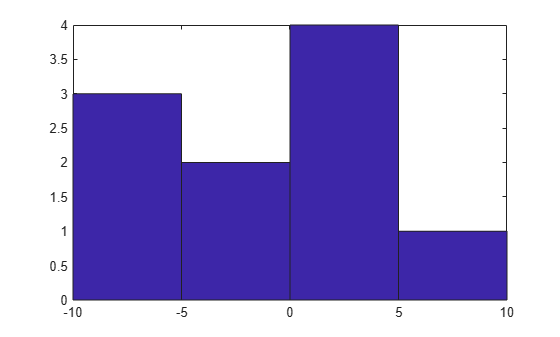## 替换不建议使用的 hist 和 histc 实例

### 旧直方图函数（`hist` 和 `histc`）

MATLAB® 早期版本使用 `hist``histc` 函数作为创建直方图和计算 bin 计数的主要方法。这些函数适用于某些常规用途，但总体能力有限。基于这些原因（及其他原因），不建议在新代码中使用 `hist``histc`

• 使用 `hist` 创建直方图后，修改该直方图的属性会有难度并要求重新计算整个直方图。

• `hist` 的默认行为是使用 10 个 bin，这对很多数据集都不适用。

• 绘制归一化直方图需要手动计算。

• `hist``histc` 的行为并不一致。

### 推荐的直方图函数

`histogram``histcounts``discretize` 函数显著提高了 MATLAB 中创建和计算直方图的能力，同时提升了一致性和易用性。要为新代码创建和计算直方图，推荐使用 `histogram``histcounts``discretize` 函数。

• `histogram` 可以返回一个直方图对象。您可以使用该对象修改直方图的属性。

• `histogram``histcounts` 都具有自动分 bin 和归一化功能，内置了几个常用选项。

• `histcounts``histogram` 的主要计算函数。这样各函数就具有一致的行为。

• `discretize` 为确定每个元素的 bin 位置提供了其他选择和灵活性。

### 要求代码更新的差异

`hist` 的代码更新

`hist` 为输入矩阵的每一列创建直方图并在同一图窗中并排绘制直方图。

```A = randn(100,2); hist(A)```

`histogram` 将整个输入矩阵视为一个高向量并创建一个直方图。要绘制多个直方图，请为每列数据创建一个不同的直方图对象。使用 `hold on` 命令在同一图窗中绘制直方图。

```A = randn(100,2); h1 = histogram(A(:,1),10) edges = h1.BinEdges; hold on h2 = histogram(A(:,2),edges) ```

Bin 设定

`hist` 接受 bin 中心作为另一个输入项。

`histogram` 接受 bin 边界作为另一个输入项。

`histogram(A,'BinLimits',[-3,3],'BinMethod','integers')`

`hist` 返回 bin 计数作为一个输出参数，也可以选择返回 bin 中心作为另一个输出参数。

```A = randn(100,1); [N, Centers] = hist(A)```

`histogram` 返回一个直方图对象作为输出参数。该对象包含许多相关属性（bin 计数、bin 边界等）。可通过更改直方图的属性值修改它的各个方面。有关详细信息，请参阅 `histogram`

```A = randn(100,1); h = histogram(A); N = h.Values Edges = h.BinEdges```

```A = randn(100,1); histogram(A) histcounts(A)```

bin 范围

`hist` 使用最小和最大有限数据值来确定绘图中第一个和最后一个条形的左边缘与右边缘。`-Inf``Inf` 分别包含在第一个和最后一个 bin 中。

```A = randi(5,100,1); histogram(A,10,'BinLimits',[min(A) max(A)])```

`histc` 的代码更新

`histc` 为输入数据的每列计算 bin 计数。对于 `m`×`n` 输入矩阵，`histc` 返回大小为 `length(edges)`×`n` 的 bin 计数矩阵。

```A = randn(100,10); edges = -4:4; N = histc(A,edges)```

`histcounts` 将整个输入矩阵视为一个高向量并计算整个矩阵的 bin 计数。

```A = randn(100,10); edges = -4:4; N = histcounts(A,edges)```

```A = randn(100,10); nbins = 10; N = zeros(nbins, size(A,2)); for k = 1:size(A,2) N(:,k) = histcounts(A(:,k),nbins); end ```

```A = 1:4; edges = [1 2 2.5 3] N = histcounts(A) N = histcounts(A,edges) ```

`histc` 中最后的 bin 主要用于整数计数。要使用 `histcounts` 执行这种整数计数，可以使用 `'integers'` bin 方法：

`N = histcounts(A,'BinMethod','integers'); `

`histc` 返回 bin 计数作为一个输出参数，也可以选择返回 bin 索引作为另一个输出参数。

```A = randn(15,1); edges = -4:4; [N,Bin] = histc(A,edges)```
• 对于 `N = histc(A,edges)``[N,bin] = histc(A,edges)` 之类的 bin 计数计算，请使用 `histcounts``histcounts` 函数返回 bin 计数作为一个输出参数，也可以选择返回 bin 边界作为另一个输出参数，或返回 bin 索引作为第三个输出参数。

```A = randn(15,1); [N,Edges,Bin] = histcounts(A)```
• 对于 `[~,Bin] = histc(A,edges)` 之类的 bin 位置计算，请使用 `discretize``discretize` 函数提供用于确定每个元素的 bin 位置的其他选项。

```A = randn(15,1); edges = -4:4; Bin = discretize(A,edges)```

#### 将 bin 中心转换为 bin 边

`hist` 函数接受 bin 中心，而 `histogram` 函数接受 bin 边界。要更新代码以使用 `histogram`，您可能需要将 bin 中心转换为 bin 边界，以重现使用 `hist` 实现的结果。

```A = [-9 -6 -5 -2 0 1 3 3 4 7]; centers = [-7.5 -2.5 2.5 7.5]; hist(A,centers)``````d = diff(centers)/2; edges = [centers(1)-d(1), centers(1:end-1)+d, centers(end)+d(end)];```

`hist` 函数包括落入每个 bin 右边界上的值（第一个 bin 包含两个边界），而 `histogram` 包括落入每个 bin 左边界上的值（最后一个 bin 包含两个边界）。稍微移动 bin 边界以获得与 `hist` 相同的 bin 计数。

`edges(2:end) = edges(2:end)+eps(edges(2:end))`
```edges = 1×5 -10.0000 -5.0000 0.0000 5.0000 10.0000 ```

`histogram(A,edges)`